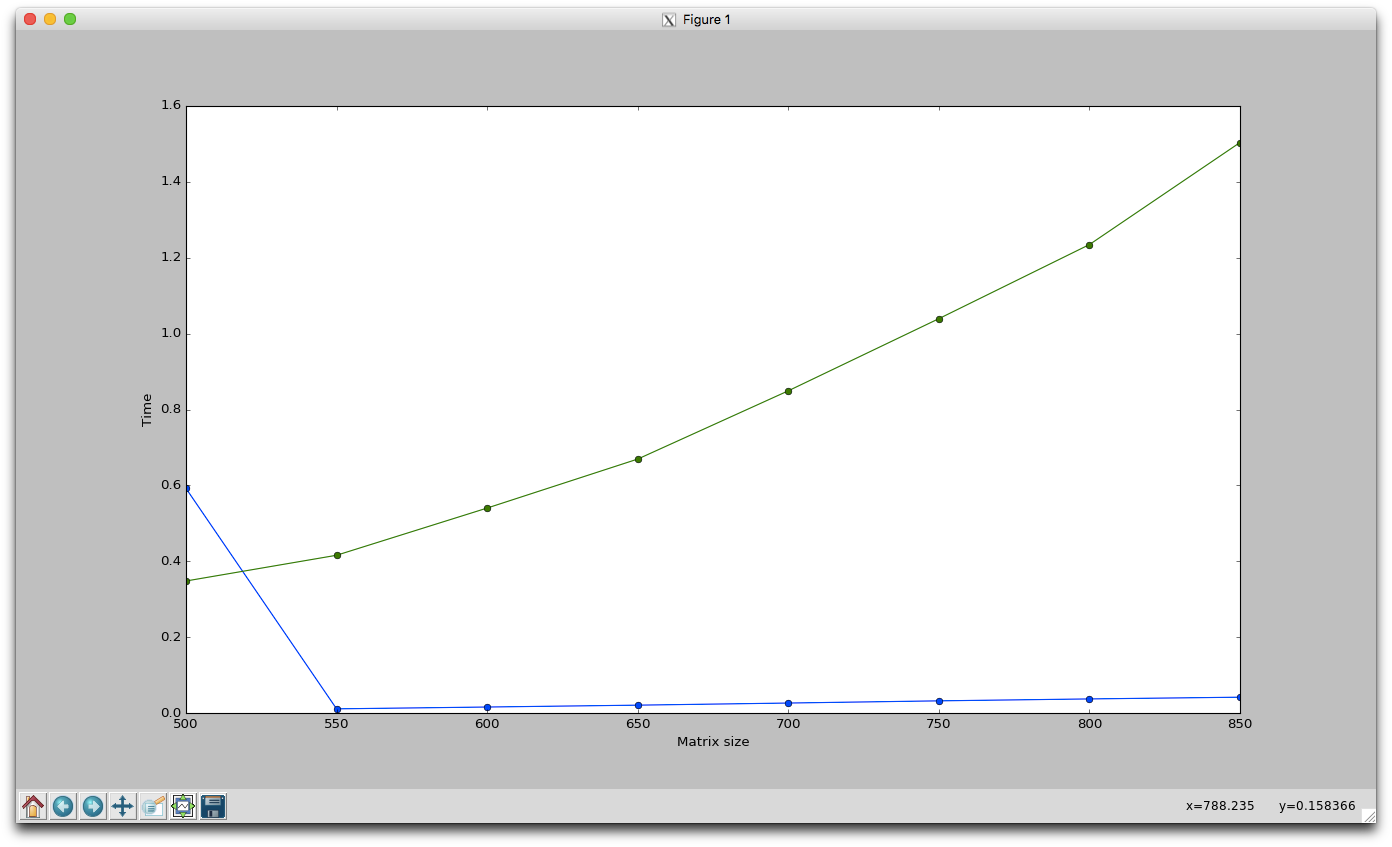# What is TensorFlow?

It’s a framework to perform computation very efficiently, and it can tap into the GPU (Graphics Processor Unit) in order too speed it up even further. This will make a huge effect as we shall see shortly. TensorFlow can be controlled by a simple Python API, which we will be using in this tutorial.

# Graphs and Tensors

When a native computation is done in many programming languages, it is usually executed directly. If you type `a = 3*4 + 2` in a Python console, you will immediately have the result. Running a number of mathematical computations like this in an IDE also allows you to set breakpoints, stop the execution and see intermediate results. This is not possible in TensorFlow, what you actually do is specifying the computations that will be done. This is accomplished by creating a computational graph, which takes multidimensional matrices called “Tensors” and does computations on them. Each node in the graph denotes an operation. When creating the graph, you have the possibility to explicitly specify where the computations should be done, on the GPU or CPU. By default it will check if a GPU is available, and use that.

# Sessions

The Graph is run in a Session, where you specify what operations to execute in the `run`-function. Data from outside may also be supplied to placeholders in the graph, so you can run it multiple times with different input. Furthermore, intermediate result (such as model weights) can be incrementally updated in variables, which will retain their values between runs.

# Example

This code example creates pairs of random matrices, clocks the multiplication of them depending on size and device placement.CPU time in green and GPU time in blue. The initial GPU delay at the first iteration is perhaps due to TensorFlow setting starting up stuff.

# Next step

In the next post we will use TensorFlow to create a recurrent neural network.

--

--